HLIBpro  2.4
Basic Matrix Algebra

# Matrix-Vector Multiplication

Matrix-vector multiplication in 𝓗𝖫𝖨𝖡𝗉𝗋𝗈 is provided in the form of a vector update: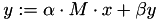Here,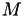is either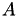,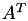or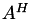for a given matrix. The individual form ofis specified by a value of type matop_t:

• apply_normal/MATOP_NORM:,
• apply_transposed/MATOP_TRANS:,
• apply_adjoint/MATOP_ADJ:.
Remarks
Since 𝓗𝖫𝖨𝖡𝗉𝗋𝗈 is based on BLAS/LAPACK, using just the conjugate of a matrix, e.g.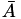, is not supported.

Each matrix classes provides the method mul_vec for real and cmul_vec for complex valued factors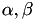(see TMatrix), which perform the corresponding operations:

unique_ptr< TVector > x( ... ); // define some vector
unique_ptr< TVector > y( ... ); // define some vector
unique_ptr< TMatrix > A( ... ); // define some matrix
A->mul_vec( 1.0, x.get(), 0.0, y.get(), apply_normal );
A->mul_vec( complex( -2.0, 0.5 ), x.get(), complex( 1.0, 4.0 ), y.get(), apply_adjoint );

Additionally, the function mul_vec is available, which also handles the case of a distributed matrix (on distributed memory). To use it, the header file algebra/mul_vec.hh has to be included.

After including algebra/mat_add.hh into the corresponding source file, the function add is available for matrix addition. It implements the matrix update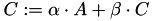with matrices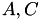. Here,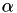and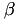are real valued. For the complex valued case, the corresponding function is called cadd:

unique_ptr< TMatrix > A( ... ); // define some matrix
unique_ptr< TMatrix > C( ... ); // define some matrix
TTruncAcc acc( 1e-6 );
-1.0, C.get(), acc );
cadd( complex( -0.5, 2.0 ), A.get(),
complex( 0.0, -1.0 ), C.get(), acc );

A crucial requirement for the matrix addition as well as for all matrix operations, is the compatibility of the corresponding index sets and cluster trees, i.e. if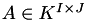then also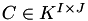must hold and the cluster trees for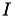and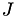have to be equal for both matrices. Only the block cluster trees may differ, e.g. a different admissibility condition can be used for both matrices.

A special for of matrix addition is implemented in add_identity (cadd_identity), in which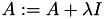is implemented:

Currently, this is only implemented ifhas dense diagonal blocks, e.g. which is th case for standard admissibility. Furthermore, since the operation only affects the diagonal part, it is an exact addition without truncation.

# Matrix Multiplication

General matrix multiplication is provided by the module mat_mul, e.g. you have to include algebra/mat_mul.hh . It is again implemented in the form of a matrix update: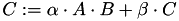Both,and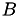may furthermore be transposed or conjugate transposed during multiplication, e.g.may be replaced byorand analogously for.

The actual multiplication is implemented in the functions multiply (for real valued) and cmultiply (for complex valued).

unique_ptr< TMatrix > A( ... ); // define some matrix
unique_ptr< TMatrix > B( ... ); // define some matrix
unique_ptr< TMatrix > C( ... ); // define some matrix
TTruncAcc acc( 1e-6 );
multiply( 2.0, apply_normal, A.get(), apply_transposed, B.get(),
-1.0, C.get(), acc );

Since matrix multiplication is computationally expensive, a progress meter may be provided to show the current state of the operation:

TConsoleProgressBar progress( cout );
multiply( 2.0, apply_normal, A.get(), apply_transposed, B.get(),
-1.0, C.get(), acc,
& progress );

As for the matrix addition, the index sets and cluster trees of the involved matrices have to be compatible, otherwise an exception is thrown during multiplication.